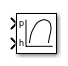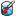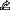# P-H Diagram (2P)

Thermodynamic scope for two-phase fluid networks

• Library:
• Simscape / Fluids / Two-Phase Fluid / Utilities

•## Description

The P-H Diagram (2P) block plots a p-h diagram of the pressure with respect to the specific enthalpy of a thermodynamic, two-phase fluid. A p-h diagram indicates the fluid state at key points during a thermodynamic cycle.

The plot units are MPa for pressure, kJ/kg for specific enthalpy, and K for the temperature contours.Each numbered point on the diagram indicates the fluid state after a dynamic process in the refrigeration cycle:

1. Cool vapor at low pressure

2. Hot vapor at high pressure

3. Hot liquid at high pressure

4. Mixed vapor and liquid at low pressure

To use the P-H Diagram (2P) block:

1. Place a Thermodynamic Properties Sensor (2P) and a Pressure & Internal Energy Sensor (2P) block at each location you want to monitor.

Note

The Pressure & Internal Energy Sensor (2P) block measures a differential pressure. Connect port A to the monitor point and port B to an Absolute Reference (2P) block.

2. Convert the Simscape physical signals to Simulink signals with a PS-Simulink Converter block.

3. Use a Mux block to combine the pressure signals. Connect the output signal to the P-H Diagram (2P) p port.

4. Use a Mux block to combine the converted specific enthalpy signals and connect the output signal to the h port.

5. Before running the simulation, double-click the P-H Diagram (2P) block, then click the Select fluid properties buttonto set the network fluid properties.

If you do not select the fluid properties, the block uses the default two-phase network fluid, which is water.

### P-H Diagram Playback

You can also replay the animation from the most recent simulation. After running the model, double-click the block and use the buttons at the top of the window to control the animation. Use the drop-down menu to the right of the Time field to control the rate of the playback. The replay controls are disabled before you run a simulation, when the model is loaded, and when the simulation is running.

Use the playback slider or field to control the simulation cycle. If you enter a time that is not a model time step, the block displays the next closest time step.

To save the current iteration of the plot, point to the plot area. From the icons in the top-right corner of the plot, click the Export iconand select the Save As buttonto save the plot.

## Ports

### Input

expand all

Vector of pressures. The vector must have same number of elements as the enthalpy signal at port h

Vector of specific enthalpies. The vector must have same number of elements as the pressure signal at port p.

## Version History

Introduced in R2021a

expand all